Courses

# Facts that Matter- Quadrilaterals Class 9 Notes | EduRev

## Mathematics (Maths) Class 9

Created by: Full Circle

## Class 9 : Facts that Matter- Quadrilaterals Class 9 Notes | EduRev

The document Facts that Matter- Quadrilaterals Class 9 Notes | EduRev is a part of the Class 9 Course Mathematics (Maths) Class 9.
All you need of Class 9 at this link: Class 9

Facts that Matter

• A plane figure bounded by four sides is called a quadrilateral.
• Sum of the angles of a quadrilateral is 360º.
• A quadrilateral is a parallelogram, if
(i) opposite sides are equal,
(ii) opposite angles are equal,
(iii) diagonals bisect each other,
(iv) a pair of opposite sides is equal and parallel.
• A diagonal of a parallelogram divides it into two congruent triangles.
• Diagonals of a rhombus bisect each other at right angles, and vice versa.
• Diagonals of a square bisect each other at right angles and are equal and vice versa.
• Diagonals of a rectangle bisect each other and vice versa.
• A line segment joining the mid-points of any two sides of a triangle is parallel to the third side.
• A line segment joining the mid-points of any two sides of a triangle is half of the third side.
• A line through the mid-point of a side of a triangle, parallel to another side bisects the third side.
• The quadrilateral formed by joining the mid-points of the sides of a quadrilateral, is a parallelogram.

A plane figure bounded by four sides is called a quadrilateral. In the following figure, four line segments AB, BC, CD and DA bound a quadrilateral ABCD.
In the given figure, we have.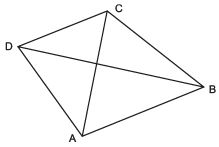(i) The points A, B, C and D are the vertices of quadrilateral ABCD.
(ii) The line segments AB, BC, CD and DA are the sides of quadrilateral ABCD.
(iii) The line segments AC and BD are called the diagonals of quadrilateral ABCD.

Note:
(i) Two sides having a common end point are called adjacent sides.
(ii) Two sides having no common end point are called opposite sides.
(iii) Two angles of a quadrilateral having a common arm are called consecutive angles.
(iv) Two angles of a quadrilateral having no common arm are called its opposite angles.

ANGLE SUM PROPERTY OF A QUADRILATERAL
The sum of all the four angles of a quadrilateral is 360º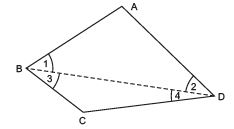Let us prove it:
In a quadrilateral ABCD, join BD. Since, the sum of the angles of a triangle is 180º.
∴ In ΔABD, we have
∠1 + ∠A + ∠2 = 180º     …(1)
In ΔBCD, we have
∠3 + ∠C + ∠4 = 180º    …(2)
Adding (1) and (2), we get
[∠1 + ∠A + ∠2] + [∠3 + ∠C + ∠4] = 180º + 180º
(∠1 + ∠3) + ∠A + ∠C + (∠2 + ∠4) = 180° + 180°
⇒ ∠B + ∠A + ∠C + ∠D = 360º
[∵ ∠1 + ∠3 = ∠B and ∠2 + ∠4 = ∠D]
∴ ∠A + ∠B + ∠C + ∠D = 360º
Thus, the sum of four angles of a quadrilateral is 360º.

(i) Parallelogram: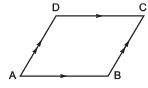A quadrilateral in which opposite sides are parallel is called a parallelogram. In the figure, ABCD is a parallelogram. Here, AB || CD and AD || BC.
Also, opposite sides of a parallelogram are equal.
(ii) Rectangle: A parallelogram, each of whose angle is 90º, is called a rectangle.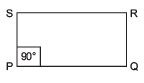In the figure, PQRS is a rectangle. We write it as rect. PQRS.
(iii) Square: A rectangle having all sides equal is called a square. In the figure, LMNO is a square.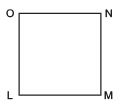(iv) Rhombus: A parallelogram having all sides equal is called a rhombus. In the figure, PQRS is a rhombus.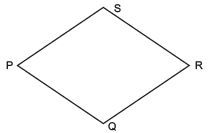(v) Trapezium: A quadrilateral in which two opposite sides are parallel and two opposite sides are nonparallel, is called a trapezium. In the figure, BCDE is a trapezium.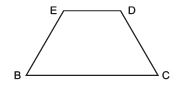Note:
If the two non-parallel sides of a trapezium are equal, then it is called an isosceles trapezium.

(vi) Kite: A quadrilateral in which two pairs of adjacent sides are equal is known as kite. PQRS is a kite such that PQ = QR and PS = RS.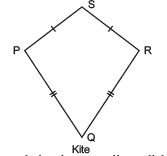Note:
(i) A square, rectangle and rhombus are all parallelograms.
(ii) A square is a rectangle and also a rhombus, but a rectangle or a rhombus is not a square.
(iii) A parallelogram is a trapezium, but a trapezium is not a parallelogram.
(iv) A kite is not a parallelogram.

PROPERTIES OF A PARALLELOGRAM
REMEMBER
1. A diagonal of a parallelogram, divides it into two congruent triangles.
2. In a parallelogram, opposite sides are equal.
3. In a parallelogram, opposite angles are equal.
4. The diagonals of a parallelogram bisect each other.

THE MID-POINT THEOREM
Statement: “The line segment joining the mid-points of any two sides of a triangle is parallel to the third side and equal to half of it.”
Proof: We have ΔABC in which D and E are the mid-points of AB and AC respectively. Let us join DE and produce it. Draw CF || BA to meet DE (produced) in F.
We have to prove that DE = (1/2)BC and DE || BC.
Now, in ΔAED and ΔCEF, we have
AE = CE   [Given]
∠AED = ∠CEF   [Vertically opposite angles]
∠DAE = ∠FCE    [∵ AB || CF and AC is a transversal]
∴ ΔAED ≌ ΔCEF  [ASA criteria]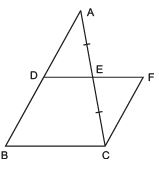⇒ Their corresponding parts are equal.
⇒ AD = CF and DE = EF
But AD = BD    [∵ D is mid point of AB]
⇒ BD = CF Also, BD || CF   [By construction]
⇒ BCFD is a parallelogram.
∴ DF = BC and DF || BC
⇒ (1/2)(DF) = (1/2)
(BC) and DE || BC    [∵ DE = EF]
⇒ DE = (1/2)
BC and DE || BC Hence, the line segment joining the mid-points of any two sides of a triangle is parallel to the third side and equal to half of it.
Converse of mid-point theorem: The line drawn through the mid-point of one side of a triangle parallel to another side, intersects the third side at its mid-point.

,

,

,

,

,

,

,

,

,

,

,

,

,

,

,

,

,

,

,

,

,

;